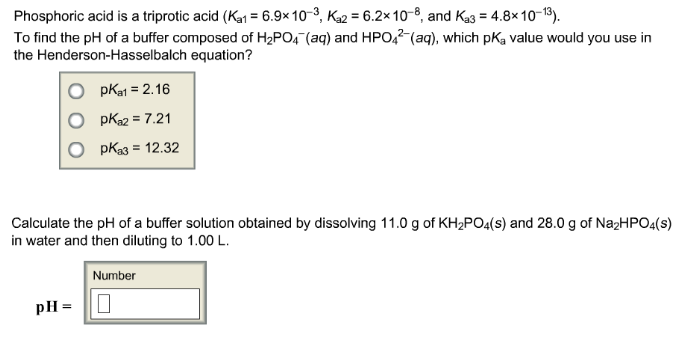# Problem: Phosphoric acid is a triprotic acid (Ka1 = 6.9 x 10-3, Ka2 = 6.2 x 10-8, and Ka3 = 4.8 x 10-13). To find the pH of a buffer composed of H2PO4- (aq) and HPO42- (aq), which pKa value would you use in the Henderson-Hasselbalch equation?                      (i) pKa1 = 2.16                      (ii) pKa2 = 7.21                      (iii) pKa3= 12.32 Calculate the pH of a buffer solution obtained by dissolving 11.0 g of KH2PO4 (s) and 28.0 g of Na2HPO4 (s) in water and then diluting to 1.00 L.

###### FREE Expert Solution
93% (293 ratings)###### Problem Details

Phosphoric acid is a triprotic acid (Ka1 = 6.9 x 10-3, Ka2 = 6.2 x 10-8, and Ka3 = 4.8 x 10-13). To find the pH of a buffer composed of H2PO4- (aq) and HPO42- (aq), which pKa value would you use in the Henderson-Hasselbalch equation?

(i) pKa1 = 2.16

(ii) pKa2 = 7.21

(iii) pKa3= 12.32

Calculate the pH of a buffer solution obtained by dissolving 11.0 g of KH2PO4 (s) and 28.0 g of Na2HPO4 (s) in water and then diluting to 1.00 L.